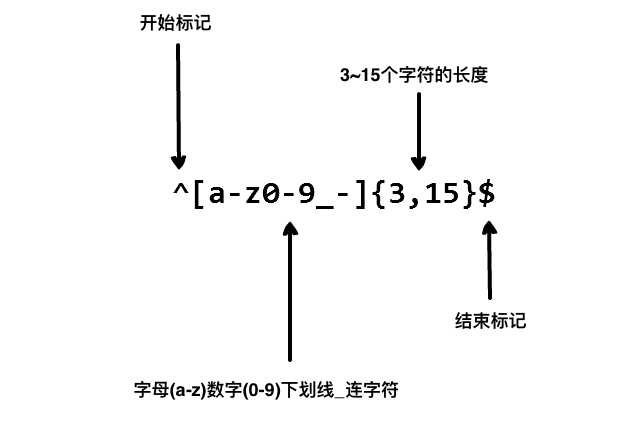# 简单高效有用的正则表达式

更新时间：2020年02月24日 18:12:34   转载 作者：ziishaned

## 什么是正则表达式？### 1. 基本匹配

```"the" => The fat cat sat on the mat.
```

```"The" => The fat cat sat on the mat.
```## 2. 元字符

. 句号匹配任意单个字符除了换行符。
[ ] 字符种类。匹配方括号内的任意字符。
[^ ] 否定的字符种类。匹配除了方括号里的任意字符
* 匹配>=0个重复的在*号之前的字符。
+ 匹配>=1个重复的+号前的字符。
? 标记?之前的字符为可选.
{n,m} 匹配num个大括号之间的字符 (n <= num <= m).
(xyz) 字符集，匹配与 xyz 完全相等的字符串.
| 或运算符，匹配符号前或后的字符.
\ 转义字符,用于匹配一些保留的字符 `[ ] ( ) { } . * + ? ^ \$ \ |`
^ 从开始行开始匹配.
\$ 从末端开始匹配.

### 2.1 点运算符 .

`.`是元字符中最简单的例子。 `.`匹配任意单个字符，但不匹配换行符。例如，表达式`.ar`匹配一个任意字符后面跟着是`a``r`的字符串。

```".ar" => The car parked in the garage.
```### 2.2 字符集

```"[Tt]he" => The car parked in the garage.
```

```"ar[.]" => A garage is a good place to park a car.
```[]好字母部分顺序，而且都是单字母，jb51就是任何包括 j或者b或者5或者1的都是可以匹配的。### 2.2.1 否定字符集

```"[^c]ar" => The car parked in the garage.
```

### 2.3.1 * 号

`*`号匹配 在`*`之前的字符出现`大于等于0`次。例如，表达式 `a*` 匹配0或更多个以a开头的字符。表达式`[a-z]*` 匹配一个行中所有以小写字母开头的字符串。

```"[a-z]*" => The car parked in the garage #21.
```

`*`字符和`.`字符搭配可以匹配所有的字符`.*``*`和表示匹配空格的符号`\s`连起来用，如表达式`\s*cat\s*`匹配0或更多个空格开头和0或更多个空格结尾的cat字符串。

```"\s*cat\s*" => The fat cat sat on the concatenation.
```

### 2.3.2 + 号

`+`号匹配`+`号之前的字符出现 >=1 次。例如表达式`c.+t` 匹配以首字母`c`开头以`t`结尾，中间跟着至少一个字符的字符串。

```"c.+t" => The fat cat sat on the mat.
```

### 2.3.3 ? 号

```"[T]he" => The car is parked in the garage.
```

```"[T]?he" => The car is parked in the garage.
```

### 2.4 {} 号

```"[0-9]{2,3}" => The number was 9.9997 but we rounded it off to 10.0.
```

```"[0-9]{2,}" => The number was 9.9997 but we rounded it off to 10.0.
```

```"[0-9]{3}" => The number was 9.9997 but we rounded it off to 10.0.
```

### 2.5 (...) 特征标群

```"(c|g|p)ar" => The car is parked in the garage.
```

### 2.6 | 或运算符

```"(T|t)he|car" => The car is parked in the garage.
```

### 2.7 转码特殊字符

```"(f|c|m)at\.?" => The fat cat sat on the mat.
```

### 2.8.1 ^ 号

`^` 用来检查匹配的字符串是否在所匹配字符串的开头。

```"(T|t)he" => The car is parked in the garage.
```

```"^(T|t)he" => The car is parked in the garage.
```

### 2.8.2 \$ 号

```"(at\.)" => The fat cat. sat. on the mat.
```

```"(at\.)\$" => The fat cat. sat. on the mat.
```

## 3. 简写字符集

. 除换行符外的所有字符
\w 匹配所有字母数字，等同于 `[a-zA-Z0-9_]`
\W 匹配所有非字母数字，即符号，等同于： `[^\w]`
\d 匹配数字： `[0-9]`
\D 匹配非数字： `[^\d]`
\s 匹配所有空格字符，等同于： `[\t\n\f\r\p{Z}]`
\S 匹配所有非空格字符： `[^\s]`
\f 匹配一个换页符
\n 匹配一个换行符
\r 匹配一个回车符
\t 匹配一个制表符
\v 匹配一个垂直制表符
\p 匹配 CR/LF（等同于 `\r\n`），用来匹配 DOS 行终止符

## 4. 零宽度断言（前后预查）

?= 正先行断言-存在
?! 负先行断言-排除
?<= 正后发断言-存在
?<! 负后发断言-排除

### 4.1 ?=... 正先行断言

`?=...` 正先行断言，表示第一部分表达式之后必须跟着 `?=...`定义的表达式。

```"(T|t)he(?=\sfat)" => The fat cat sat on the mat.
```

### 4.2 ?!... 负先行断言

```"(T|t)he(?!\sfat)" => The fat cat sat on the mat.
```

### 4.3 ?<= ... 正后发断言

```"(?<=(T|t)he\s)(fat|mat)" => The fat cat sat on the mat.
```

### 4.4 ?<!... 负后发断言

```"(?<!(T|t)he\s)(cat)" => The cat sat on cat.
```

## 5. 标志

i 忽略大小写。
g 全局搜索。
m 多行修饰符：锚点元字符 `^` `\$` 工作范围在每行的起始。

### 5.1 忽略大小写（Case Insensitive）

```"The" => The fat cat sat on the mat.
```

```"/The/gi" => The fat cat sat on the mat.
```

### 5.2 全局搜索（Global search）

```"/.(at)/" => The fat cat sat on the mat.
```

```"/.(at)/g" => The fat cat sat on the mat.
```

### 5.3 多行修饰符（Multiline）

```"/.at(.)?\$/" => The fat
cat sat
on the mat.
```

```"/.at(.)?\$/gm" => The fat
cat sat
on the mat.
```

## 6. 贪婪匹配与惰性匹配（Greedy vs lazy matching）

`"/(.*at)/" => The fat cat sat on the mat. `

`"/(.*?at)/" => The fat cat sat on the mat. `